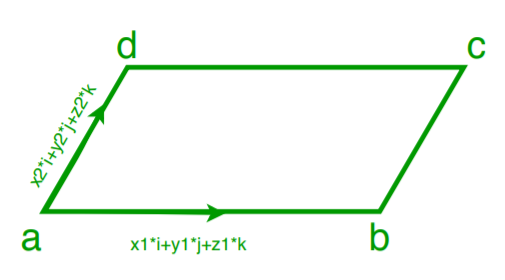# Find area of parallelogram if vectors of two adjacent sides are given

Given two vectors in form of (xi+yj+zk) of two adjacent sides of a parallelogram. The task is to find out the area of a parallelogram.

Example:

Input:
x1 = 3, y1 = 1, z1 = -2
x2 = 1, y2 = -3, z2 = 4

Output: Area = 17.3205081
Input:
x1 = 1, y1 = 3, z1 = 2
x2 = 1, y2 = -3, z2 = 4

Output: Area = 19.078784028338912

## Recommended: Please try your approach on {IDE} first, before moving on to the solution.Approach: Suppose we have two vectors a(x1*i+y1*j+z1*k) and b(x2*i+y2*j+z2*k) and we know that area of parallelogram is given by :

Area of parallelogram = magnitude of cross product of vectors a and b i.e |axb|
And we know a X b = (y1*z2 – y2*z1)*i – (x1*z2 – x2*z1)*j + (x1*y2 – x2*y1)*k

Then area =## C++

 // C++ code to calculate area of   // parallelogram if vectors of  // 2 adjacent sides are given     #include   using namespace std ;      // Function to calculate area of parallelogram    float area(float x1, float y1, float z1, float x2,              float y2, float z2)      {           float area = sqrt(pow((y1 * z2 - y2 * z1),2)                          + pow((x1 * z2 - x2 * z1),2) +                           pow((x1 * y2 - x2 * y1),2));          return area;      }          // Driver Code   int main()   {       float x1 = 3;      float y1 = 1;      float z1 = -2;      float x2 = 1;      float y2 = -3;      float z2 = 4;      float a = area(x1, y1, z1, x2, y2, z2);      cout << "Area = " << a;      return 0;   // This code is contributed   // by Amber_Saxena.  }

## Java

 // Java code to calculate area of    // parallelogram if vectors of   // 2 adjacent sides are given      public class GFG {             // Function to calculate area of parallelogram         static float area(float x1, float y1, float z1, float x2,                   float y2, float z2)           {                float area =(float) Math.sqrt(Math.pow((y1 * z2 - y2 * z1),2)                               + Math.pow((x1 * z2 - x2 * z1),2) +                                Math.pow((x1 * y2 - x2 * y1),2));               return area;           }           // Driver code       public static void main (String args[]){           float x1 = 3;               float y1 = 1;               float z1 = -2;               float x2 = 1;               float y2 = -3;               float z2 = 4;               float a = area(x1, y1, z1, x2, y2, z2);               System.out.println("Area = " + a) ;                   }     // This code is contributed by ANKITRAI1  }

## Python

 # Python code to calculate area of   # parallelogram if vectors of  # 2 adjacent sides are given     import math     # to calculate area of parallelogram   def area(x1, y1, z1, x2, y2, z2):      area = math.sqrt((y1 * z2 - y2 * z1) ** 2             + (x1 * z2 - x2 * z1) ** 2 +             (x1 * y2 - x2 * y1) ** 2)      return area     # main function  def main():      x1 = 3     y1 = 1     z1 = -2     x2 = 1     y2 = -3     z2 = 4     a = area(x1, y1, z1, x2, y2, z2)      print("Area = ", a)     # driver code      if __name__=="__main__":      main()

## C#

 // C# code to calculate area of   // parallelogram if vectors of   // 2 adjacent sides are given   using System;     class GFG   {     // Function to calculate area  // of parallelogram   static float area(float x1, float y1,                    float z1, float x2,                     float y2, float z2)   {       float area = (float) Math.Sqrt(Math.Pow((y1 * z2 - y2 * z1), 2) +                                      Math.Pow((x1 * z2 - x2 * z1), 2) +                                      Math.Pow((x1 * y2 - x2 * y1), 2));       return area;   }      // Driver code   public static void Main ()  {      float x1 = 3;       float y1 = 1;       float z1 = -2;       float x2 = 1;       float y2 = -3;       float z2 = 4;       float a = area(x1, y1, z1, x2, y2, z2);       Console.Write("Area = " + a) ;  }  }     // This code is contributed  // by ChitraNayal

## PHP

 

Output:

Area =  17.320508075688775


Attention reader! Don’t stop learning now. Get hold of all the important DSA concepts with the DSA Self Paced Course at a student-friendly price and become industry ready.

My Personal Notes arrow_drop_upCheck out this Author's contributed articles.

If you like GeeksforGeeks and would like to contribute, you can also write an article using contribute.geeksforgeeks.org or mail your article to contribute@geeksforgeeks.org. See your article appearing on the GeeksforGeeks main page and help other Geeks.

Please Improve this article if you find anything incorrect by clicking on the "Improve Article" button below.